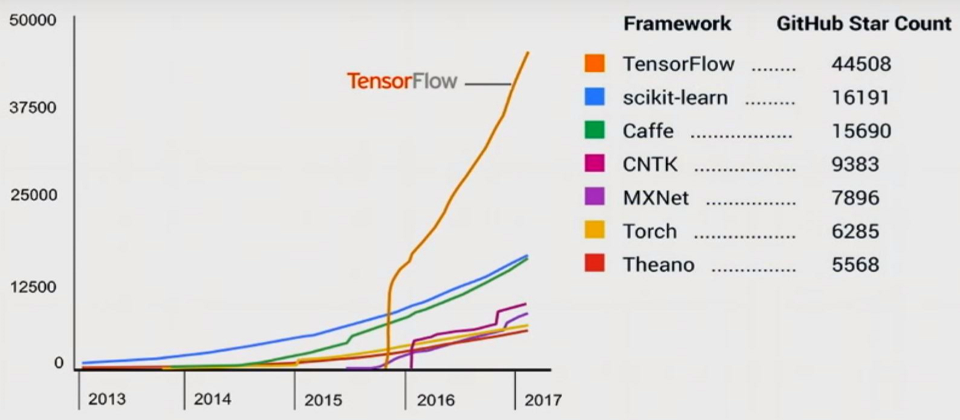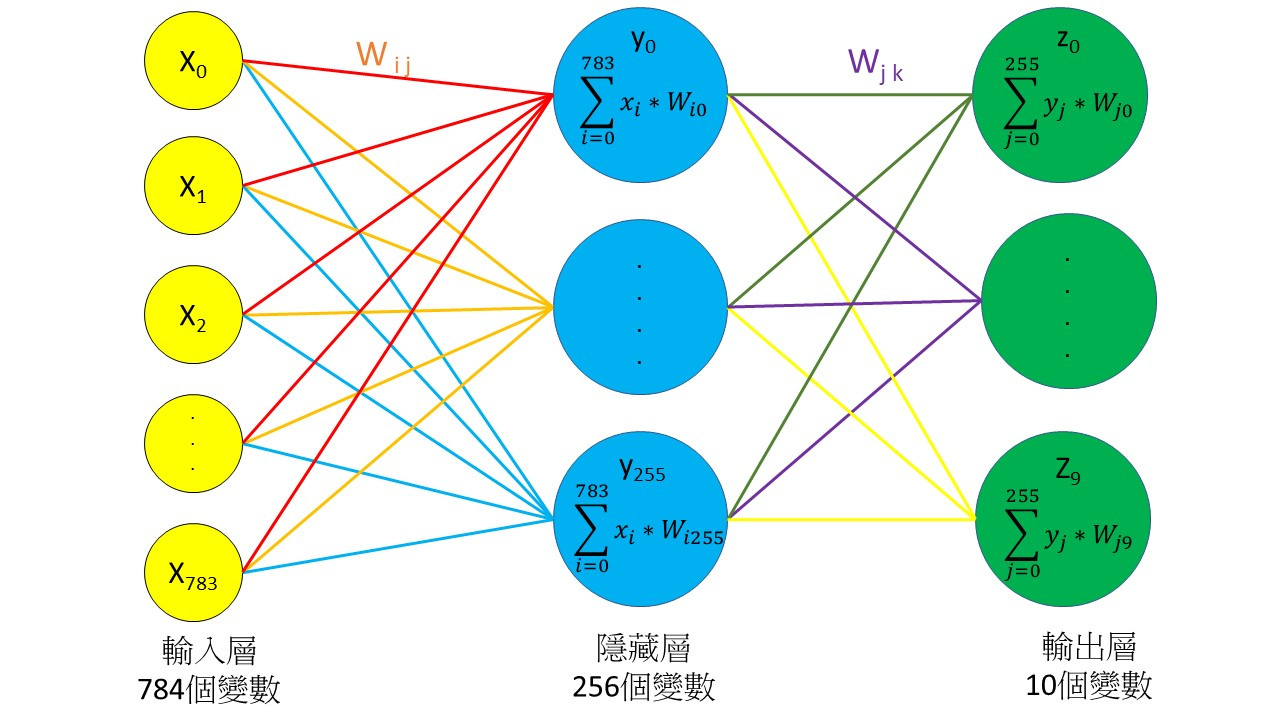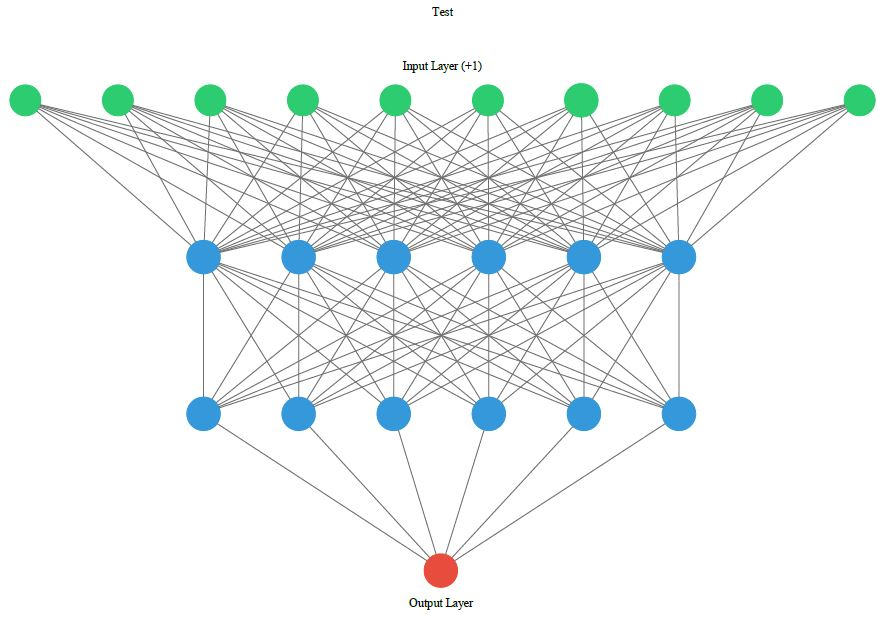# Neural Network

在〈Neural Network〉中留言功能已關閉

tensorflow是深度學習的一個框架，目前最多人使用。其實深度學習的框架蠻多的，比如PyTorch就是另一個蠻常看到的框架。支援 Python 的Neural Network 框架請見下圖說明。# Keras

Keras 凱拉斯，是支援 TensorFlow 的更高階函數庫，可以用簡潔的程式碼完成一個Neural Network模型。

# 什麼神經網路x 跟 y 之間的每一條連線，都是一個權重值，y 跟 z 之間的連線也是權重。

$y_{0}=\sum_{i=0}^{i=n}x_{i}*W_{i0}$
$y_{1}=\sum_{i=0}^{i=n}x_{i}*W_{i1}$
…………………………..

$z_{0}=\sum_{j=0}^{j=2}y_{j}*W_{j0}$
$z_{1}=\sum_{j=0}^{j=2}y_{j}*W_{j1}$

todo

# 模型視覺化

Add Graphviz to system PATH for all user

sudo apt-get install graphviz

pip install ann-visualizer graphviz tensorflow

from keras.models import Sequential;
from keras.layers import Dense;
network = Sequential();
#Hidden Layer#1
activation='relu',
kernel_initializer='uniform',
input_dim=11));
#Hidden Layer#2
activation='relu',
kernel_initializer='uniform'));
#Exit Layer